## 2.04.2012

### Trigonometric table tangent cotangent in degrees

Trigonometric table tangent cotangent in degrees - it is the four-valued table of tangents and cotangents in degrees within one minute. A few examples are made at the beginning, as to use this table. For comfort in a table additional navigation elements are plugged with pointing by the name of the names of functions and values of minutes column-wise for every function. Value of degrees and minutes for every trigonometric function distinguished by different colors: for tangents - green, for cotangents - blue. Connotations for minutes are distinguished by yellow.

A trigonometric table for tangents and cotangents consists of two parts. The first part includes tangents from 0 to 75 degrees and cotangents from 15 to 90 degrees. Before to use this table, it is recommended on present examples to find the values of tangents and cotangents on a table and compare results. If all turned out correctly for you, means you can consider itself an experience user of table.At the use it is necessary to remember this table, that additional values one, two and three minutes for a tangent have a sign plus and at addition added and at deduction subtracted, for a cotangent they have a sign minus and at addition subtracted, and at deduction added. For verification compare the got result to the values of the same name trigonometric functions in the alongside located cells.

The second part of trigonometric table includes tangents from 75 to 90 degrees and cotangents from 0 to 15 degrees. To use this table some simpler, than by the first part.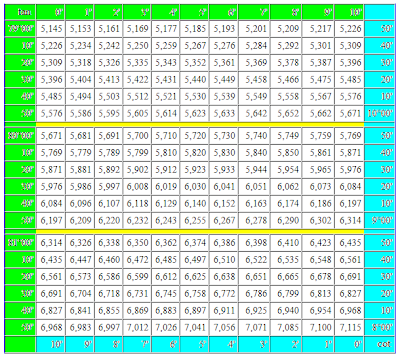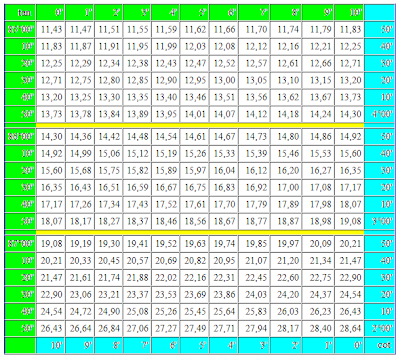For a tangent 90 degrees and cotangent 0 degrees a value is not certain. It is so accepted to consider because task on dividing by a zero mathematicians until now so not succeeded to decide.

If you need a trigonometric table of sines and cosines, then she can be found on a separate page.

## 1.10.2012

### Trigonometric table of sines and cosines

The trigonometric table of values of sines and cosines within one minute is counted on blondes. For comfort of the use for sines and cosines distinguished this table of value of corners by different colors. For sines the blue color of cells is accepted with degrees and by minutes. For cosines the green background of cells is accepted. A yellow background is distinguish the values of minutes that if necessary is added or subtracted from tabular values.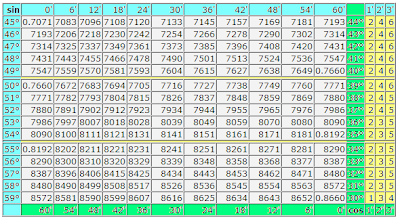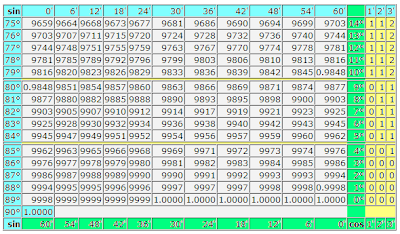Usually it is not accepted so in detail to give a navigation on a trigonometric table. Firstly, a table is counted on experience users by mathematics. Secondly, publishers from old times produce these tables in a blackly white variant and in every way save printer's ink on a navigation on a trigonometric table.

I hope, such registration will not give to lose way you even in the middle of this table and you will not entangle sines with cosines at the search of their values. By the way, the trigonometric table of sines and cosines presents the values of these trigonometric functions for corners from 0 to 90 degrees. For other values of corners can use a trigonometric circle as a crib.

If you need more exact calculation of values of trigonometric functions, then can take advantage of calculator. How to use the table of Брадиса, we will understand next time. Specially for those, whoever knows.

## 11.08.2011

### Table of squares of natural numbersIn the table of squares of natural numbers the squares of numbers are presented from 1 to 100. Index of exponent of number as a number two writtenin on the right in the top of that number that is involuted. If a number is erected in a square, it means that this number needs to be increased on a such is exact number.

For example, ten in a square equals one hundred, because if ten to multiplication on ten, for us will turn out one hundred. Five in the second degree equals twenty five, because all know that five at five "again twenty five". And two in a square it not that another, "as twice two four".

Why was such thought of is a exponent of number? For short of record of mathematical expressions, whatever to repeat several times multiplication of identical numbers. If at erection of number in the second exponent we save a quite small place, then at erection of numbers in great exponents the economy of place turns out rather significant.

By the feature of squares of numbers, as well as all other even degrees of number, there is that after involution negative number a positive number ensues. In fact minus on minus gives a plus at an multiplication, how many times we this focus did not repeat. A negative number is in the second, fourth, sixth, eighth et cetera degrees gives a positive number as a result.

## 10.27.2011

### Multiplication and division by zero

As a zero is not a number, all mathematical operations on multiplying and dividing by a zero take place in area of units of measurements. In relation to the operation of divizion by zero of unit of measuring can be real and virtual. Units of measurements of length behave to the real units of measurements. All other units of measurements, probably, are virtual. Dividing by the zero of virtual units of measuring is impossible, as a result of division by zero of such units of measuring does not make sense.

In the special group it is necessary to distinguish natural unit of measurement of speeds (speed of light) and mathematical unit of corners (corner in 45 degrees). These units of measurements hatch through mathematical methods and their mathematical properties require a further study. More detailed study is required similarly by units of measurements of time.

Virtual units of measurements appear as a result of process that mathematically can be written down as dividing of zero by zero.

0/0=1а

where а – is virtual unit of measurement.

The described mathematical properties of virtual units of measurements allow to enter any units of measurements us and use them without influence on the surrounding world. These units of measurements are used both for description of surrounding reality and for everyday needs. The examples of virtual units of measurements can be units of measurements of money, temperature, many physical sizes or applied in a technique and commerce. The process of exit from everyday life of virtual units of measurements of measuring can be mathematically represented as multiplying by a zero. Mathematical properties of similar units of measurements are tested by practice of their use during many millenniums.

In multidimensional space, division by zero increases the amount of the spatial dimensions, multiplcanion by zero diminishes this amount.

In rectangular cartesian coordinates it will look so:

x/0 = xy
xy/0 = (x/0)y = x(y/0) = xyz

At multiplication by zero it is necessary to take into account project properties of space, as a result of such increase depends on that, which one component is multiplied by zero.

xyz*0 = 0 and xy or xz or yz
xy*0 = 0 and x or y

In physical equalizations, division by zero requires introduction of new unit of measurement to examined by equalization the physical co-operation expressed by a mathematical action by an increase (probably, another unit of measurement of length). For example, if to divide unit of measurement of length into a zero, unit of measurement of area will ensue. If to divide unit of measurement of area into a zero, unit of measurement of volume will ensue et cetera.

m/0 = m²
m²/0 = m³

Algebraically it can be presented in a next kind:

a/0 = ab
ab/0 = abc

where а, b, c - mutually perpendicular units of measurements of length.

At multiplication by a zero one of the components of co-operation, described by physical equalization, from co-operation is eliminated. The primary result of co-operation grows into a zero. Remaining components continue to co-operate.

m³*0 = 0 and
m²*0 = 0 and m

Algebraically it can be presented in a next kind:

abc*0 = 0 and ab or ac or bc
ab*0 = 0 and a or b

where а, b, c - mutually perpendicular units of measurements of length.

Expl for blondes: It only began division and multiplication by zero. More in detail we will consider it other time.

## 10.26.2011

### Space evolution

Probably, in the process of the evolution, space generates different multidimensional universes with the even amount of dimension. Development originates from spaces with less of dimension to spaces with plenty of dimension. For basis of existence of space it is possible to accept principle of existence of speeds. Our 6-dimension Universe in the chain of space evolution will look like the following.Quite possible, that to Big Bang putting beginning of our Universe, there was a 4-dimension universe in that energy had one dimension of length, and a matter two dimension of length in that part of universe that corresponds our slower-than-light. A black hole, the consequence of that in 6-dimension space was Big Bang, giving beginning of our Universe, appeared in the process of evolution of this 4-dimension universe. A matter with two dimension of length in our Universe grew into 2-dimensional energy. Question about transformation of unidimensional on length energy in the process of transition through a black hole, remains open.

In the process of evolution of our Universe energy with two dimension of length partly passes to the matter with three dimension of length. A matter generates in our Universe black holes that give beginning to the new universes in the eightmeasured space. After Big Bang in the eightmeasured space our matter with three measuring of length grows into energy of the eightmeasured universe. Et cetera. The process of space evolution can develop for ever and ever.

During an evolution one 4-dimension universe generates the great number of 6-dimension universes. In turn every 6-dimension universe generates the great number of the 8-dimension universes. It look like the process of spawning. All 6-dimension universe, generated by one 4-dimension universe, can be in the mutually perpendicular dimension, that eliminates their cross-coupling on each other. Each of universes is mapped to all other universes as a point. The 8-dimension universes can be formed just.

In regard to faster-than-light part of universes of any type the theory of symmetry deserves attention in relation to velocity of light. Energy and matter of slower-than-light part freely move in three dimension of length and hardly fixed in the continuous stream of three dimension of time. Dark energy and dark matter freely move in three dimension of time and hardly fixed in the continuous stream of three dimension of length. Even if it not so, possibility of existence of universes of similar type does not need to be thrown down from accounts.

A transition process from a black hole to Big Bang requires an additional study. Mathematically he can be described by the operations of multiplication and division by zero. There are grounds to suppose that the trigger mechanism of gravitational collapse, resulting in appearance of black hole, is space geometry. This question will be considered additionally.

### Trigonometric dependences of corner of scale

We will consider trigonometric dependences of corner of scale in two rectangular triangles - at the increase of scale and at diminishing of scale.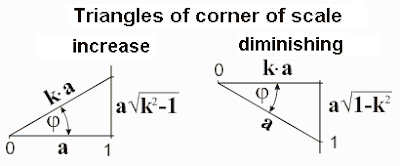Trigonometric correlations of parties of the got rectangular triangles for diminishing and increase of scale we will take in a table.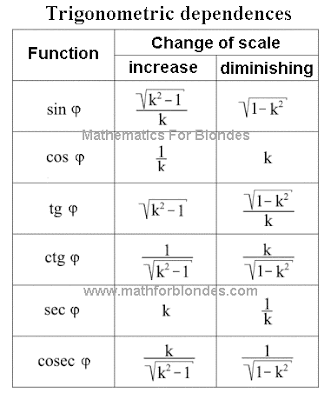The got results can be compared to the relativistic radical from the theory of relativity of Einstein. Radicals in these equalizations very look like a sine and secant of diminishing of corner of scale. If to execute not difficult transformations, it is possible to get unit of measurement.The conducted transformations specify on that natural unit of measurement of speeds is speed of light. There are no grounds to suppose that there is a change of trigonometric dependences at passing through the point of symmetry of coefficient of scale. On it, it is possible to suppose that for faster-than-light speeds a relativistic radical takes on next values.On the basis of foregoing it is possible to suggest a next hypothesis about the structure of our Universe. Speed of set is a natural barrier separating slower-than-light part of Universe from faster-than-light part. Slower-than-light part of Universe we have possibility to look after. By virtue of specific properties of velocity of light, faster-than-light part of Universe can not be observed directly. It is possible to suppose that velocity of light is the axis of symmetry of distribution of substance in Universe. Dark matter and dark energy, that render affecting our part of Universe, can be in faster-than-light part of Universe.

If in Universe there are highly developed reasonable civilizations that captured faster-than-light technologies, then for an information transfer they will use not hertzian waves possessing velocity of light, and hard carriers of information on the basis of dark matter, transmissible with speeds, considerably excelling velocity of light.

If to equate trigonometric dependences of corner of scale with the values of trigonometric functions at 90° (it 1; 0 and 1/0), then for diminishing of scale they are taken to equality 0=1, for the increase of scale - to equality of k=0.

Our Universe has three limitations. In space by the border of universe явля-ется area, where velocity of light equals a zero. Outwardly our Universe is a point in space. Mathematical equalization of universe is equality 0=1 - any physical quantity with the unit of measurement in the scales of Universe equals a zero. This law of maintenance is confirmed by some researches of physicists, in particular, about it talked in the lecture of Andrei Linde.

Except spatial limitation, I exist limitations of speed. In slower-than-light part of Universe it is expressed in limitation long - distance between two positions of any point of space in time can not equal a zero. In physics this limitation it is accepted to name the absolute pitch of temperature. Faster-than-light part of Universe has limitation at times - time between two positions of any point in space can not equal a zero. The instantaneous moving in space without moving in time is impossible. Geometrically it can be expressed so: projection of speed on length and for a time can not equal a zero. Implementation of these terms is provided by the presence of rotation on the most different levels: atomic, planetary, galactic. It is possible to suppose that our Universe is similarly revolved in space.

More exact idea about principles of existence of Universe it is possible to get after the detailed study velocities of light as a physical process. For basis it is possible to accept position that velocity of light in our Universe is the result of co-operation of three dimension length with three dimension of time. Mathematically this co-operation is described by an multiplication. Physically our Universe has six dimension - three dimension of length and three dimension of time.

Expl for blondes: Now time to draw our Universe to look, what place she occupies in the space evolution.

## 10.25.2011

### Change of corner of scale

The increase of corner of scale can be presented as a change of quantity at unchanging unit of measurement. Diminishing of corner of scale can be presented as a change of unit of measurement at an unchanging quantity. Geometrically in the system of rectangular triangle it will look like the following.An increase and diminishing of scale in the identical amount of one times correspond to one value of corner of scale. On this property of corners of scale trigonometric dependences are based in a rectangular triangle.

From the point of view of mathematical result does not matter, as a change of quantity is described in relation to unit of measurement. A variable quantity at permanent unit of measurement and variable unit of measurement at a permanent quantity will give the identical value of corner of scale.

In a general view the change of scale of quantity can be presented as a turn of unit of measurement on the size of corner of scale. An increase or diminishing of scale depends on that, what position of unit of measurement is taken for basis at comparison and from the type of projection. The increase of scale can be presented as a radial projection on a number ray, perpendicular to the unit of measurement taken for basis at comparison. Diminishing of scale can be presented as a perpendicular projection of the compared unit of measurement on the unit of measurement taken for basis.Expl for blondes: A boring entry is farther closed and interesting begins are trigonometric dependences of corner of scale.